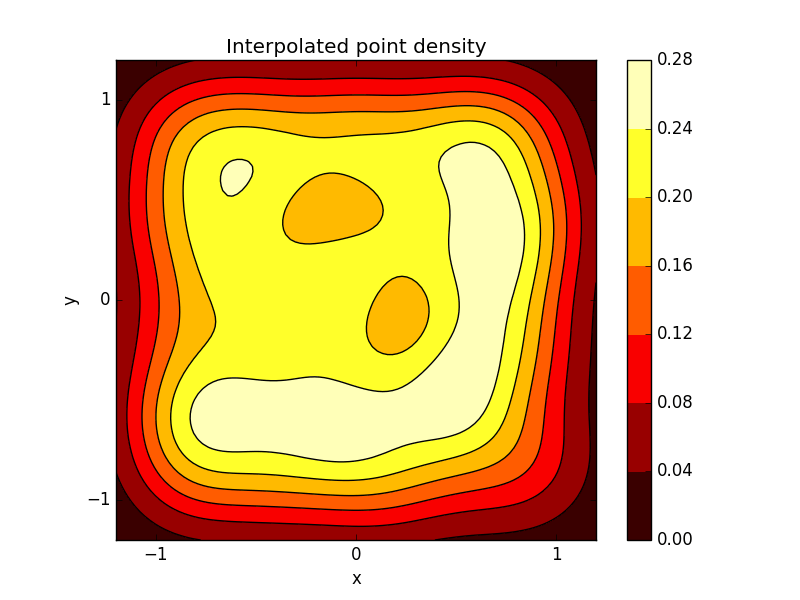# Introduction to plotting with Python and Matplotlib

Here is a brief example of how to plot data with Python and, specifically, the matplotlib library.

The following program calculates an estimate of Pi by generating random points in the range [-1;1) x [-1;1), and using the ratio between the number of points inside the unit circle to the total number of points. There are, of course, far more efficient methods to compute Pi, but here we are more concerned with the visualization of this process – namely, the plotting.

import numpy as np  # Python mathematics library

N = 500             # Number of points
xlim = -1.2, 1.2    # X-axis range for plot
ylim = -1.2, 1.2    # Y-axis range for plot
ticks = -1, 0, 1    # Ticks to show on plot

#### Compute random points in [-1, 1) x [-1, 1)

X,Y = 2*np.random.rand(2, N) - 1

#### Compute Pi

# Create array for colours
colours = ['b' for _ in range(N)]

# Counter for points inside unit circle
count = 0

# Calculare pi and plot points
for i in range(N):
point = X[i], Y[i]
if np.linalg.norm(point) <= 1:
count += 1
colours[i] = 'r'

mypi = 4*count/N
print("Pi is:", mypi)
print("Error:", mypi-np.pi)


You will also notice that we are populating an array called colours. If a point is inside the unit circle, its corresponding entry in colours is 'r', otherwise it is 'b'. This will be used in the next step, when we use matplotlib to generate a scatter plot of our points, also showing which ones are inside the unit circle and which ones aren’t:

import matplotlib.pyplot as plt

#### Scatter plot

# Plot unit circle
fig, ax = plt.subplots()
t = np.linspace(0, 2*np.pi, 100)
ax.plot(np.cos(t), np.sin(t), 'k')

# Plot points
ax.scatter(X, Y, c=colours)

# Plot's aesthetics
ax.set_aspect('equal')
ax.set_title('Points used to calculate Pi')
ax.set_xlabel('x')
ax.set_ylabel('y')
ax.set_xlim(xlim)
ax.set_ylim(ylim)
ax.set_xticks(ticks)
ax.set_yticks(ticks)

# Save figure
fig.savefig('scatter.pdf')
plt.close(fig)


Here you can see that the colours array is passed to the .scatter() method, along with the coordinates of the points.
Matplotlib supports a variety of formats, including PDF, PNG and JPEG.Next, something a little more fancy. We use the scipy library to calculate the interpolated point density of our scatter plot, and then generate a contour plot to visualise it.

from scipy import stats

#### Create contour plot

xmin, xmax = xlim
ymin, ymax = xlim

# Calculate interpolation
_X, _Y = np.mgrid[xmin:xmax:100j, ymin:ymax:100j]
positions = np.vstack([_X.ravel(), _Y.ravel()])
values = np.vstack([X, Y])
kernel = stats.gaussian_kde(values)
Z = np.reshape(kernel(positions), _X.shape)

# Plot data
fig, ax = plt.subplots()
mappable = ax.contourf(Z, extent=(*xlim,*ylim), cmap=plt.get_cmap('hot'))
ax.contour(Z, extent=(*xlim,*ylim), colors='k')

fig.colorbar(mappable)

# Plot's aesthetics
ax.set_aspect('equal')
ax.set_title('Interpolated point density')
ax.set_xlabel('x')
ax.set_ylabel('y')
ax.set_xlim(xlim)
ax.set_ylim(ylim)
ax.set_xticks(ticks)
ax.set_yticks(ticks)

# Save file
fig.savefig('contour.pdf')
plt.close()


contourf supports many colour maps, for instance hot, coolwarm or winter. A complete reference can be found here.